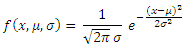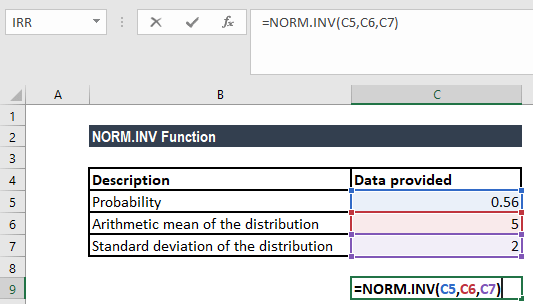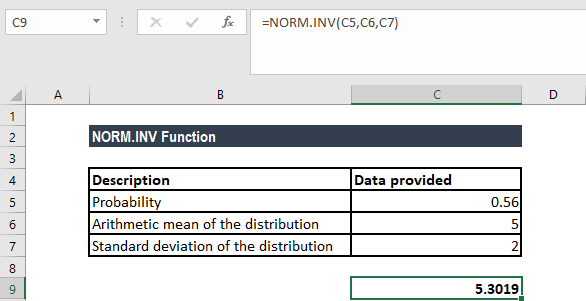# NORM.INV Function

Calculate the inverse of the normal cumulative distribution for a given value of x

## What is the NORM.INV Function?

The NORM.INV Function is categorized under Excel Statistical functions. It will calculate the inverse of the normal cumulative distribution for a supplied value of x, with a given distribution mean and standard deviation. The function will calculate the probability to the left of any particular point in a normal distribution.

For example, suppose we are given a normally distributed random variable that is denoted by x. For the value of x, if we wish to get the bottom 5% of the distribution, we can use the NORM.INV function.

As a financial analyst, the function is useful in stock market analysis. We can use NORM.INV to understand how a portfolio is affected by any additions or withdrawals made.

### Formula

=NORM.INV(probability,mean,standard_dev)

The NORM.INV formula uses the following arguments:

1. Probability (required argument) – This is the probability corresponding to normal distribution. It is the value at which we want to evaluate the inverse function.
2. Mean (required argument) – This is the arithmetic mean of the distribution.
3. Standard_dev (required argument) – This is the standard deviation of the distribution.

The normal distribution is a continuous probability function and is given by the formula:Where:

• μ is the mean of the distribution
• σis the variance
• is the independent variable for which you want to evaluate the function

### How to use the NORM.INV Function in Excel?

The NORM.INV function was introduced in Excel 2010. To understand the uses of the function, let’s consider an example:

#### Example

Suppose we are given the following data:

• Probability: 0.56
• Arithmetic mean of the distribution: 5
• Standard deviation of the distribution: 2

If we wish to calculate the value of the function, the formula to use will be:We get the result below:### Notes about the NORM.INV Function

1. If the mean equals zero and the standard deviation equals 1, NORM.INV uses the standard normal distribution.
2. #VALUE! error – Occurs if any of the given arguments is non-numeric.
3. #NUM! error – Occurs when:
• The given probability argument is less than zero or greater than one.
• The given standard_dev argument is less than or equal to zero.

Thanks for reading CFI’s guide to important Excel functions! By taking the time to learn and master these functions, you’ll significantly speed up your financial modeling and analysis. To learn more, check out these additional CFI resources:

• Excel Finance Functions